# DMRG algorithm for the Ising model in a transverse field¶

The goal of this exercise is to implement the finite version of the DMRG algorithm for the Ising model in a transverse field (TFIM) for a chain of spins one-half and study the model close to the quantum phase transition.

The Hamiltonian is: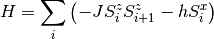where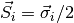are the spin operators, andare the Pauli matrices.

This model has a quantum phase transition at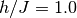. At the critical point the exact value for the ground state energy for a finite system with open boundary conditions is given by: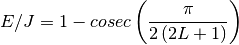The high field phase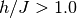is a paramagnet with an order parameter. The low field phase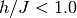is a ferromagnet with an order parameter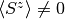. At the critical point, both order parameters go to zero.

The exact values of the energies at the critical point are:

L E/L (J)
16 -1.2510242438
20 -1.255389856
32 -1.2620097863
40 -1.264235845
64 -1.267593439

## Exercise¶

Study the quantum phase transition in the TFIM by measuring the order parameters at each side of the transition and comparing the energy at the critical point with the exact result. The critical point is

• calculate the energy per site for a given system size and different number of states kept, make a plot, and extrapolate the values of the energy to zero truncation error with a linear fit. Compare this with the exact value for a finite size given by the exact solution.
• measure the value of the order parameters for a given system size and a few values of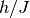around the critical point. Use a reasonable system size and a number of states, so you actually are able to get to sample the phase diagram around the critical point. Get the order parameter by summing up the values of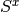orfor all sites of the chain. Plot both order parameters versus.

## Solution¶

The implementation goes is pretty similar to the one for the Heisenberg model of the last exercise. The main change is of course the change of the Hamiltonian, block Hamiltonian, and the operators you need to update after each DMRG step.

To simplify things and switching between different models, the System class has a few convience functions to do grow the blocks, perform the infinite and finite DMRG steps, and set the Hamiltonian. These are the same functions we have seen before, but now written as methods of the System class.

When using these methods you have to write a Model class that contains the details of the TFIM model:

class TranverseFieldIsingModel(object):
"""Implements a few convenience functions for the TFIM.

Does exactly that.
"""
def __init__(self, h = 0):
super(TranverseFieldIsingModel, self).__init__()
self.h = h

def set_hamiltonian(self, system):
"""Sets a system Hamiltonian to the TFIM Hamiltonian.

Does exactly this. If the system hamiltonian has some other terms on
it, there are not touched. So be sure to use this function only in
newly created System objects.

Parameters
----------
system : a System.
The System you want to set the Hamiltonain for.
"""
system.clear_hamiltonian()
if 'bh' in system.left_block.operators.keys():
if 'bh' in system.right_block.operators.keys():

def set_block_hamiltonian(self, system):
"""Sets the block Hamiltonian to be what you need for TFIM.

Parameters
----------
system : a System.
The System you want to set the Hamiltonian for.
"""
# If you have a block hamiltonian in your block, add it
if 'bh' in system.growing_block.operators.keys():

def set_operators_to_update(self, system):
"""Sets the operators to update to be what you need to TFIM.

Parameters
----------
system : a System.
The System you want to set the Hamiltonian for.
"""
# If you have a block hamiltonian in your block, update it
if 'bh' in system.growing_block.operators.keys():


The best thing is to look at the final implementation and compare to the previous one for the Heisenberg model:

def main(args):
#
# create a system object with spin one-half sites and blocks, and set
# its model to be the TFIM.
#
spin_one_half_site = SpinOneHalfSite()
system = System(spin_one_half_site)
system.model = TranverseFieldIsingModel()
#
# read command-line arguments and initialize some stuff
#
number_of_sites = int(args['-n'])
number_of_states_kept = int(args['-m'])
number_of_sweeps = int(args['-s'])
system.model.H = float(args['-H'])
number_of_states_infinite_algorithm = 10
if number_of_states_kept < number_of_states_infinite_algorithm:
number_of_states_kept = number_of_states_infinite_algorithm
sizes = []
energies = []
entropies = []
truncation_errors = []
system.number_of_sites = number_of_sites
#
# infinite DMRG algorithm
#
max_left_block_size = number_of_sites - 3
for left_block_size in range(1, max_left_block_size+1):
energy, entropy, truncation_error = (
system.infinite_dmrg_step(left_block_size,
number_of_states_infinite_algorithm) )
current_size = left_block_size + 3
sizes.append(left_block_size)
energies.append(energy)
entropies.append(entropy)
truncation_errors.append(truncation_error)
#
# finite DMRG algorithm
#
states_to_keep = calculate_states_to_keep(number_of_states_infinite_algorithm,
number_of_states_kept,
number_of_sweeps)
half_sweep = 0
while half_sweep < len(states_to_keep):
# sweep to the left
for left_block_size in range(max_left_block_size, 0, -1):
states = states_to_keep[half_sweep]
energy, entropy, truncation_error = (
system.finite_dmrg_step('right', left_block_size, states) )
sizes.append(left_block_size)
energies.append(energy)
entropies.append(entropy)
truncation_errors.append(truncation_error)
half_sweep += 1
# sweep to the right
# if this is the last sweep, stop at the middle
if half_sweep == 2 * number_of_sweeps - 1:
max_left_block_size = number_of_sites / 2 - 1
for left_block_size in range(1, max_left_block_size + 1):
energy, entropy, truncation_error = (
system.finite_dmrg_step('left', left_block_size, states) )
sizes.append(left_block_size)
energies.append(energy)
entropies.append(entropy)
truncation_errors.append(truncation_error)
half_sweep += 1
#
# save results
#
output_file = os.path.join(os.path.abspath(args['--dir']), args['--output'])
f = open(output_file, 'w')
zipped = zip (sizes, energies, entropies, truncation_errors)
f.write('\n'.join('%s %s %s %s' % x for x in zipped))
f.close()
print 'Results stored in ' + output_file


See a full implementation of the above code. To learn how to run this code you can use:

\$ ./solutions/tfim.py --help
Implements the full DMRG algorithm for the S=1/2 TFIM.

Calculates the ground state energy and wavefunction for the
Ising model in a transverse field for a chain of spin one-half. The
calculation of the ground state is done using the full DMRG algorithm,
i.e. first the infinite algorithm, and then doing sweeps for
convergence with the finite algorithm.

Usage:
tfim.py (-m=<states> -n=<sites> -s=<sweeps> -H=<field>) [--dir=DIR -o=FILE]
tfim.py -h | --help

Options:
-h --help         Shows this screen.
-n <sites>        Number of sites of the chain.
-m <states>       Number of states kept.
-s <sweeps>       Number of sweeps in the finite algorithm.
-H <field>        Magnetic field in units of coupling between spins.
-o --output=FILE  Ouput file [default: tfim.dat]
--dir=DIR         Ouput directory [default: ./]